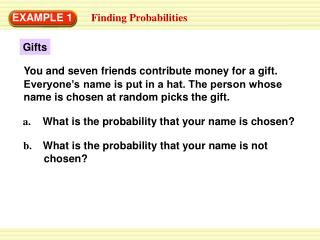Download PresentationEXAMPLE 1

# EXAMPLE 1 - PowerPoint PPT PresentationDownload Presentation## EXAMPLE 1

- - - - - - - - - - - - - - - - - - - - - - - - - - - E N D - - - - - - - - - - - - - - - - - - - - - - - - - - -
##### Presentation Transcript

1. You and seven friends contribute money for a gift. Everyone’s name is put in a hat. The person whose name is chosen at random picks the gift. EXAMPLE 1 Finding Probabilities Gifts a. What is the probability that your name is chosen? b. What is the probability that your name is not chosen?

2. Number of favorable outcomes = Number of possible outcomes 1 = 8 1 – 1 = 8 7 = 8 EXAMPLE 1 Finding Probabilities SOLUTION a.P(your name is chosen) b. P(your name is not chosen) =1 –P(your name is chosen).

3. EXAMPLE 2 Finding Odds Vacation Survey You do a survey asking your class torank three vacation choices. Results for “the beach” are shown at the right. What are the odds in favor of a randomly chosen student from your class ranking a beach vacation first?

4. Number of favorable outcomes = Number of unfavorable outcomes 6 = 21 2 = 7 EXAMPLE 2 Finding Odds SOLUTION The beach was ranked first by 6 students, so there are 6 favorable outcomes. It was ranked second by 12students, and ranked third by 9 students, so there are 12 + 9 =21 unfavorable outcomes. Odds in favor

5. ANSWER The odds in favor of a randomly chosen student ranking a beach vacation first are2to7. EXAMPLE 2 Finding Odds

6. 3 1.P(A)= 4 ANSWER 1 4 for Examples 1 and 2 GUIDED PRACTICE You are given the probability that event A will occur. Find the probability that event Awill not occur.

7. ANSWER 0.55 for Examples 1 and 2 GUIDED PRACTICE You are given the probability that event A will occur. Find the probability that event Awill not occur. 2. P(A)= 0.45

8. ANSWER = 68% for Examples 1 and 2 GUIDED PRACTICE You are given the probability that event A will occur. Find the probability that event Awill not occur. 3. P(A)= 32%

9. 7 4. P(A)= 10 ANSWER 3 = 10 for Examples 1 and 2 GUIDED PRACTICE You are given the probability that event A will occur. Find the probability that event Awill not occur.

10. 5. The 11 letters in the word MISSISSIPPI are each written on pieces of paper and randomly chosen from a bag. What is the probability of drawing an Sfrom the bag? What is the probability of not drawing an S? ANSWER The probability of drawing an Sfrom the bag 4 = 11 The probability of not drawing an S 7 = 11 for Examples 1 and 2 GUIDED PRACTICE

11. ANSWER 1 = 23 for Examples 1 and 2 GUIDED PRACTICE You choose a card at random from a set of cards numbered 1 to 24. Find the odds in favor of the event. 6. You choose a 10.

12. ANSWER = 1 for Examples 1 and 2 GUIDED PRACTICE 7. You choose an odd number.

13. ANSWER 1 = 2 for Examples 1 and 2 GUIDED PRACTICE 8. You choose an even number greater than8.